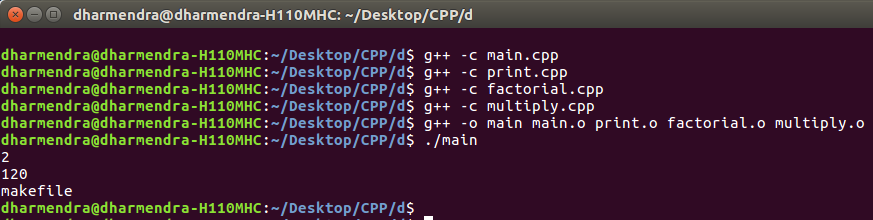# MakeFile in C++ and its applications

Given a C++ program and the task is to break the entire program in the form of Makefile.
It is basically used to create .cpp file and .h file for each class/functions and its functionalities and later link them all through a Makefile command available in C++.

• It makes codes more concise and clear to read and debug.
• No need to compile entire program every time whenever you make a change to a functionality or a class. Makefile will automatically compile only those files where change has occurred.
• Generally, in long codes or projects, Makefile is widely used in order to present project in more systematic and efficient way.

Example: Create a program to find the factorial and multiplication of numbers and print it.

 `// Program to calculate factorial and ` `// multiplication of two numbers. ` `#include ` `using` `namespace` `std; ` ` `  `// Function to find factorial ` `int` `factorial(``int` `n) ` `{ ` `    ``if` `(n == 1) ` `        ``return` `1; ` `    ``// Recursive Function to find  ` `    ``// factorial ` `    ``return` `n * factorial(n - 1); ` `} ` ` `  `// Function to multiply two numbers ` `int` `multiply(``int` `a, ``int` `b) ` `{ ` `    ``return` `a * b; ` `} ` ` `  `// Function to print ` `void` `print() ` `{ ` `    ``cout << ``"makefile"` `<< endl; ` `} ` ` `  `// Driver code ` `int` `main() ` `{ ` `    ``int` `a = 1; ` `    ``int` `b = 2; ` `    ``cout << multiply(a, b) << endl; ` `    ``int` `fact = 5; ` `    ``cout << factorial(5) << endl; ` `    ``print(); ` `    ``return` `0; ` `} `

Output:

```2
120
makefile```

Use Makefile to run the above program:

• File Name: main.cpp

 `#include ` ` `  `// Note function.h which has all functions ` `// definations has been included ` `#include "function.h" ` ` `  `using` `namespace` `std; ` ` `  `// Main program ` `int` `main() ` `{ ` `    ``int` `num1 = 1; ` `    ``int` `num2 = 2; ` `    ``cout << multiply(num1, num2) << endl; ` `    ``int` `num3 = 5; ` `    ``cout << factorial(num3) << endl; ` `    ``print(); ` `} `

• File Name: print.cpp

 `#include ` ` `  `// Definition of print function is ` `// present in function.h file ` `#include "function.h" ` `using` `namespace` `std; ` ` `  `void` `print() ` `{ ` `    ``cout < ``"makefile"` `<< endl; ` `} `

• File Name: factorial.cpp

 `#include ` ` `  `// Definition of factorial function ` `// is present in function.h file ` `#include "function.h" ` `using` `namespace` `std; ` ` `  `// Recursive factorial program ` `int` `factorial(``int` `n) ` `{ ` `    ``if` `(n == 1) ` `        ``return` `1; ` `    ``return` `n * factorial(n - 1); ` `} `

• File Name: multiply.cpp

 `#include ` ` `  `// Definition of multiply function  ` `// is present in function.h file ` `#include "function.h" ` `using` `namespace` `std; ` ` `  `int` `multiply(``int` `a, ``int` `b) ` `{ ` `    ``return` `a * b; ` `} `

• File Name: functions.h

 `#ifndef FUNCTIONS_H ` `#define FUNCTIONS_H ` ` `  `void` `print(); ` `int` `factorial(``int``); ` `int` `multiply(``int``, ``int``); ` ` `  `#endif `

Commands to Compile and Run above program:

```Open Terminal and type commands:
g++ -c main.cpp
g++ -c print.cpp
g++ -c factorial.cpp
g++ -c multiply.cpp
g++ -o main main.o print.o factorial.o multiply.o
./main

Note: g++ -c filename.cpp is used to create object file.
```My Personal Notes arrow_drop_upCheck out this Author's contributed articles.

If you like GeeksforGeeks and would like to contribute, you can also write an article using contribute.geeksforgeeks.org or mail your article to contribute@geeksforgeeks.org. See your article appearing on the GeeksforGeeks main page and help other Geeks.

Please Improve this article if you find anything incorrect by clicking on the "Improve Article" button below.

Article Tags :
Practice Tags :

2

Please write to us at contribute@geeksforgeeks.org to report any issue with the above content.